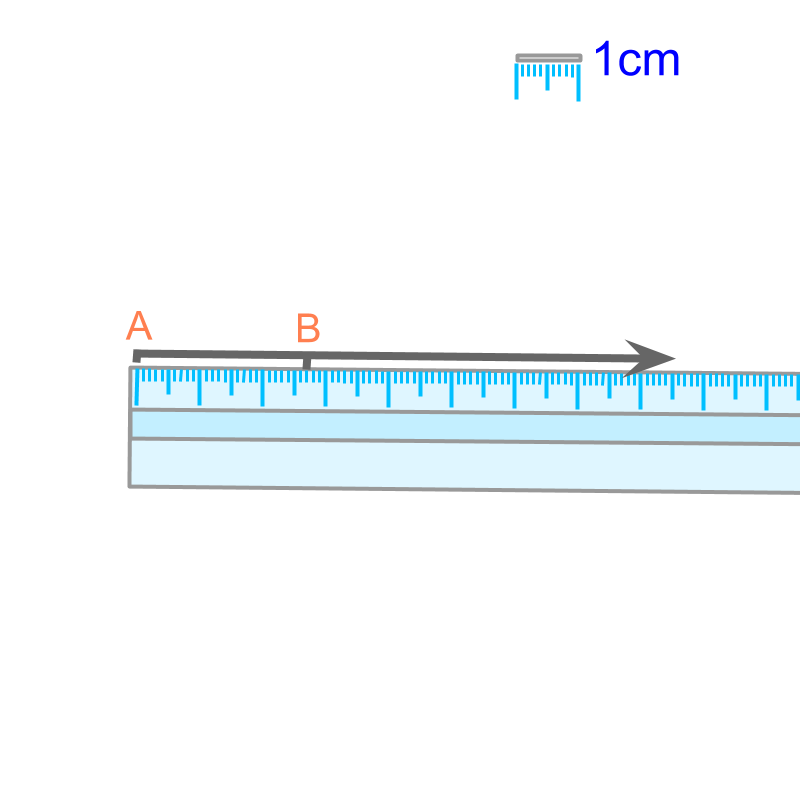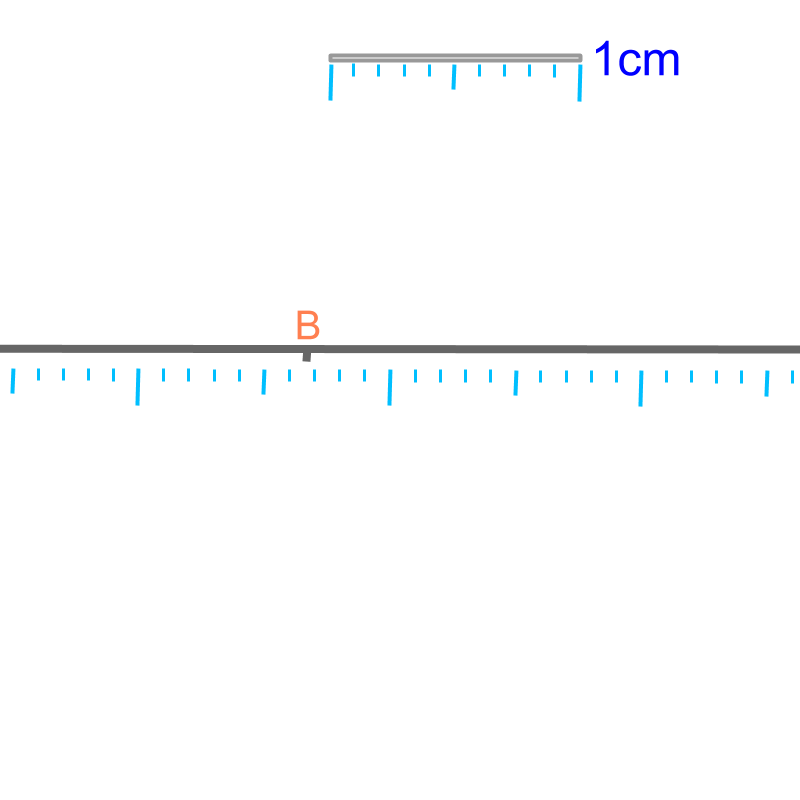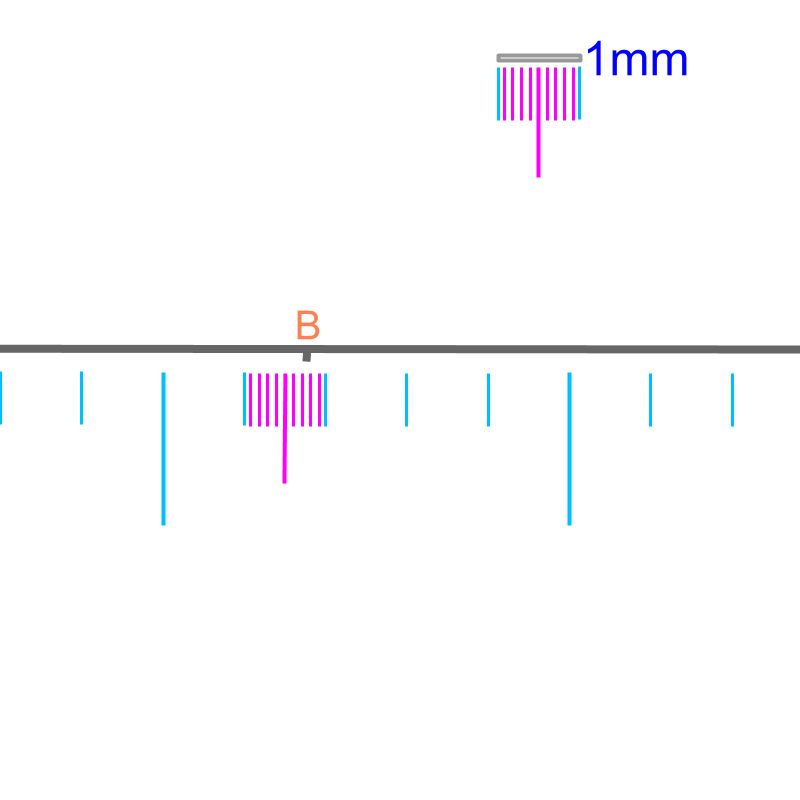maths > mensuration-basics

Accurate measure and Approximate measure

what you'll learn...

Overview

Accuracy of Measurements : Measurement can be performed to a desired accuracy level.

When the accuracy is specified to a lower level, the measurement is approximate measurement.

exact

Consider a pencil. How to measure the length of a pencil?
We can directly measure the distance-span between the two ends of the pencil. OR, we can mark the ends of the pencil onto a line-segment and measure the length of the line-segment.To measure the length of a pencil, an equivalent line segment is formed. The line segment and the graduated scale are shown in the figure. Note the legend on the right-top of the figure showing $1$$1$ centimeter. The length of the line segment $\overline{AB}$$\overline{A B}$ is "$2.7$$2.7$ cm".The figure is magnified. Note the legend showing the modified $1$$1$ cm length. The point $A$$A$ is not shown. It is noted that, the length of $\overline{AB}$$\overline{A B}$ is approximately $2.7$$2.7$ cm.The figure is magnified even larger. Note the legend showing the modified $1mm$$1 m m$ length. As per the figure, the length of $\overline{AB}$$\overline{A B}$ is better approximated to $2.78$$2.78$ cmThe line-segment $\overline{AB}$$\overline{A B}$ can be measured at different accuracies.

•  The length is $2.7$$2.7$ cm when measured with a graduated-scale.

•  The length is $2.78$$2.78$ cm when magnified and measured with a better instrument than a scale.

•  The length is $2.78892\cdots$$2.78892 \cdots$ when measured at much higher accuracy. For most practical applications, the length of $2.7$$2.7$ cm is good enough.

Note 1: The line represents a real object. It can be a leaf, or a rod. The important point being, the measurements in real-life are approximated.

Note 2: The length of a real object can be exactly measured too. For example, a pencil of length exactly $2$$2$cm is possible. That object, when magnified and measured with high accuracy, will be of length $2$$2$cm only.

Note 3: The length of a real object can be a decimal number, that does not end or does not repeat. For example, a pencil is of length $3.78023\cdots$$3.78023 \cdots$cm length. The decimals in the number does not end or repeat. It is an interesting observation, that will help to understand some concepts later.

The word "accuracy" means: state of being exactly correct.

summary

Accuracy of Measurements : Measurement can be performed to a desired accuracy level.

When the accuracy is specified to a lower level, the measurement is approximate measurement.

Outline

The outline of material to learn "Mensuration basics : Length, Area, & Volume" is as follows.

•  Measuring Basics

→   Introduction to Standards

→   Measuring Length

→   Accurate & Approximate Meaures

→   Measuring Area

→   Measuring Volume

→   Conversion between Units of Measure

•  2D shapes

→   Perimeter of Polygons

→   Area of Square & rectangle

→   Area of Triangle

→   Area of Polygons

→   Perimeter and area of a Circle

→   Perimeter & Area of Quadrilaterals

•  3D shapes

→   Surface Area of Cube, Cuboid, Cylinder

→   Volume of Cube, Cuboid, Cylinder